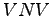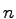## 3. 4. 4 Conclusion

The only topological requirement on the finite difference method is that a neighborhood of vertices can be found for each single vertex. This topological function for retrieving all neighboring vertices of a given vertex is denoted as traversal function.

It also has to be mentioned that the neighboring vertices of a given vertex can be found much easier, if a structured grid is given. However, a finite difference method can also be applied, if no further topological information is available. For instance, the neighborhood can be defined by the closestpoints of a given point.

If the topological information is given, one can chose to find the neighborhood by finding all incident points which are covered by an edge or a cell incident to the original vertex. It is also possible to use more vertices by choosing more neighboring cells by further applying the incidence relation such as for higher order finite difference schemes on structured grids .

Michael 2008-01-16